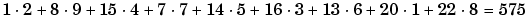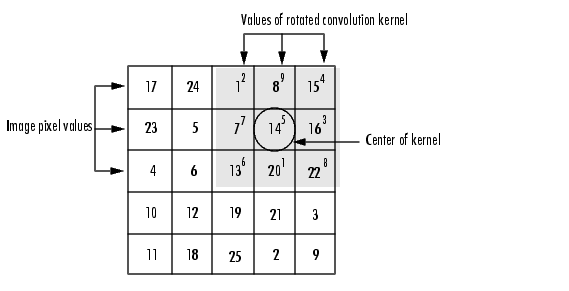# OpenCV Convolution (filter2D) Does Not RotatePosted in software by Christopher R. Wirz on Sat Dec 22 2018

﻿

Some libraries produce image convolutions assuming the kernel is rotated 180 degrees - OpenCV does not. This post will quickly show that OpenCV does not rotate the image using a quick C++ implementation.

Note: This post assumes OpenCV 3 (which includes previous version OpenCV 2).

First, let's take a look at MATLAB's documentation on convolutions...

## Convolution

Linear filtering of an image is accomplished through an operation called convolution. Convolution is a neighborhood operation in which each output pixel is the weighted sum of neighboring input pixels. The matrix of weights is called the convolution kernel, also known as the filter. A convolution kernel is a correlation kernel that has been rotated 180 degrees.

For example, suppose the image is

```A = [17  24   1   8  15
23   5   7  14  16
4   6  13  20  22
10  12  19  21   3
11  18  25   2   9]
```

and the convolution kernel is

```h = [8   1   6
3   5   7
4   9   2]
```

The following figure shows how to compute the (2,4) output pixel using these steps:

1. Rotate the convolution kernel 180 degrees about its center element.
2. Slide the center element of the convolution kernel so that it lies on top of the (2,4) element of A.
3. Multiply each weight in the rotated convolution kernel by the pixel of A underneath.
4. Sum the individual products from step 3.
Hence the (2,4) output pixel isSo let's try this in OpenCV using C++...

``````
#include <opencv2/opencv.hpp>
#include <iostream>

/// <summary>
///		Prints the matrix.
/// </summary>
/// <param name="mat">The matrix (pointer).</param>
void printMat(cv::Mat* mat)
{
printf("(%dx%d)\n", mat->cols, mat->rows);
for (int i = 0; i < mat->rows; i++)
{
if (i == 0)
{
for (int j = 0; j < mat->cols; j++)  printf("%10d", j + 1);
}

printf("\n%4d: ", i + 1);
for (int j = 0; j < mat->cols; j++)
{
printf("%10.2f", mat->at<float>(i, j));
}
}
printf("\n");
}

/// <summary>
///		The main function
/// </summary>
/// <param name="argc">The argument count.</param>
/// <param name="argv">The argument vector.</param>
/// <returns>0 for success</returns>
int main(int argc, char** argv)
{
// Create the original matrix
// CV_32FC1 means "32 bit float, 1 channel"
// Note: sizeof(float) = 4 and 4*8 = 32
cv::Mat original = cv::Mat(3, 3, CV_32FC1);

// Create the kernel
cv::Mat filter = cv::Mat(3, 3, CV_32FC1);

// Set everything to 0
for (int r = 0; r < 3; r++) {
for (int c = 0; c < 3; c++) {
original.at<float>(r, c) = 0.0;
filter.at<float>(r, c) = 0.0;
}
}

// Specify the bottom right = 1.0 for testing
original.at<float>(2, 2) = 1.0;
filter.at<float>(2, 2) = 1.0;

cv::Mat filtered;

// Convolve a matrix with the kernel
filter2D(original, filtered,
-1, // -1 for same as source
filter, // the filter just specified
cv::Point(-1, -1), // cv::Point(-1, -1) is the center
0, // value added to each pixel
cv::BORDER_ISOLATED // do not look outside of ROI
);

printf("Starting matrix:\n");
printMat(&original);
printf("\nFilter matrix:\n");
printMat(&filter);
printf("\nFiltered matrix:\n");
printMat(&filtered);
return 0;
}
```
```

Here's the results:

``````
Starting matrix:
(3x3)
1         2         3
1:       0.00      0.00      0.00
2:       0.00      0.00      0.00
3:       0.00      0.00      1.00

Filter matrix:
(3x3)
1         2         3
1:       0.00      0.00      0.00
2:       0.00      0.00      0.00
3:       0.00      0.00      1.00

Filtered matrix:
(3x3)
1         2         3
1:       0.00      0.00      0.00
2:       0.00      1.00      0.00
3:       0.00      0.00      0.00
```
```

What does this mean? This implies the calculation for the middle element is

``````
1         2         3
1:       0.00      0.00      0.00
2:       0.00      0.00      0.00
3:       0.00      0.00      1.00 * 1.00
```
```

Given that the image matrix is

``````
1         2         3
1:       0.00      0.00      0.00
2:       0.00      0.00      0.00
3:       0.00      0.00      1.00
```
```

and the filter matrix is

``````
1         2         3
1:       0.00      0.00      0.00
2:       0.00      0.00      0.00
3:       0.00      0.00      1.00
```
```

It it should be clear that OpenCV does not rotate the convolution kernel.
If so, the calculation would look like

``````
1              2         3
1:       0.00* 1.00  0.00      0.00
2:       0.00        0.00      0.00
3:       0.00        0.00      1.00 * 0.00
```
```

Which would produce a matrix of all zeros.